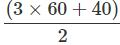Courses

# Test: Time - 3

## 20 Questions MCQ Test Mathematics (Maths) for Class 4 | Test: Time - 3

Description
This mock test of Test: Time - 3 for Class 4 helps you for every Class 4 entrance exam. This contains 20 Multiple Choice Questions for Class 4 Test: Time - 3 (mcq) to study with solutions a complete question bank. The solved questions answers in this Test: Time - 3 quiz give you a good mix of easy questions and tough questions. Class 4 students definitely take this Test: Time - 3 exercise for a better result in the exam. You can find other Test: Time - 3 extra questions, long questions & short questions for Class 4 on EduRev as well by searching above.
QUESTION: 1

### How many weeks are there in 1 year?

Solution:

There are 52 weeks in a year.

QUESTION: 2

### How many days were there in February 2003?

Solution:

2003 is not a leap year. So, February has 28 days in 2003.

QUESTION: 3

### Which of these years was a leap year?

Solution:

Of the given years, 1996 is exactly divisible by 4 and hence is a leap year.

QUESTION: 4

How many months are there in 4 years 3 months?

Solution:

4 years 3 months =(4 ×12 + 3) months = 51 months

QUESTION: 5

How many days are there in 5 weeks 4 days?

Solution:

5 weeks 4 days = 39 days

QUESTION: 6

How many hours are there in the month of April?

Solution:

April has 30 days. Number of hours in April =30 × 24 = 720 hours

QUESTION: 7

How many minutes make a quarter of an hour?

Solution:

1/4 * 60 = 15 minutes

QUESTION: 8

What time is it 2 hours before midnight?

Solution:

12 p.m.− 2 hours = 10 p.m.

QUESTION: 9

Meena takes 7 minutes 20 seconds to sew 8 buttons. How long does Meena take to sew one button?

Solution:

Time taken to sew 8 buttons = 7 minutes 20 seconds =(7 × 60 + 20) seconds = 440 seconds
∴ Time taken to sew 1 button = 440/8 second = 55 second

QUESTION: 10

How many months in a year have 31 days?

Solution:
QUESTION: 11

How many weeks are there in 147 days?

Solution:
QUESTION: 12

Sumanth was born in 1976. How old was he in 1993?

Solution:
QUESTION: 13

ATV film starts at 5:30 p. m. on a Sunday. It runs for 2 hours 15 minutes. At what time does it end?

Solution:
QUESTION: 14

How many minutes are there in a day?

Solution:
QUESTION: 15

Find the sum of 6 years 8 months and 3 years 6 months.

Solution:

6 years 8 months + 3 years 6 months = 80 months + 42 months = 122 months

QUESTION: 16

Shiela reached the library at 12:05 p.m. The bus ride to the library lasted 45 minutes. At what time did Shiela board the bus?

Solution:

Time at which Shiela reached the library = 12:05 p.m. Duration of bus journey = 45 minutes.
∴ The time at which she boarded the bus =12:05 p.m.−45 minutes=11:20 a.m.

QUESTION: 17

Jai spent 3 hours 40 minutes over two days on his homework. If he spent the same amount of time each day, how much time was spent per day?

Solution:

Total time spent on two days for homework = 3 hours 40 minutes The same amount of time is spent on each of the 2 days.
∴ Time spent per day on homework = 3 hours 40 minutes ÷ 2 =∴ 110 minutes = (60 + 50) minutes = 1 hour 50 minutes.

QUESTION: 18

Mallesh sells 380 eggs per day. How many eggs would he sell in half a year? (1 year = 52 weeks)

Solution:

No. of eggs sold per day = 380
∴ No. of eggs sold per week =380 × 7 = 2660 1 year = 52 weeks 1/2 year = 52/2 weeks = 26 weeks
∴ No. of eggs sold in 26 weeks = 2660 × 26 eggs = 69160 eggs

QUESTION: 19

A train leaves town P at 12:05 p.m. At what time will it reach town Q, if the journey takes 1 hour and 42 minutes?

Solution:

12:05 p.m.+ 1 h 42 min = 1:47 p.m.

QUESTION: 20

Hema ran a race in 109 seconds. She ran 12 seconds faster than Chandni. How long did Chandni take to run the race?

Solution:

No. of seconds Hema took to run the race = 109 seconds = 12 seconds faster than that of Chandni
∴ The time taken by Chandni to run the race  = (109 + 12) seconds = 121 seconds = 2 minutes 1 second (Since 60 seconds = 1 minute.)